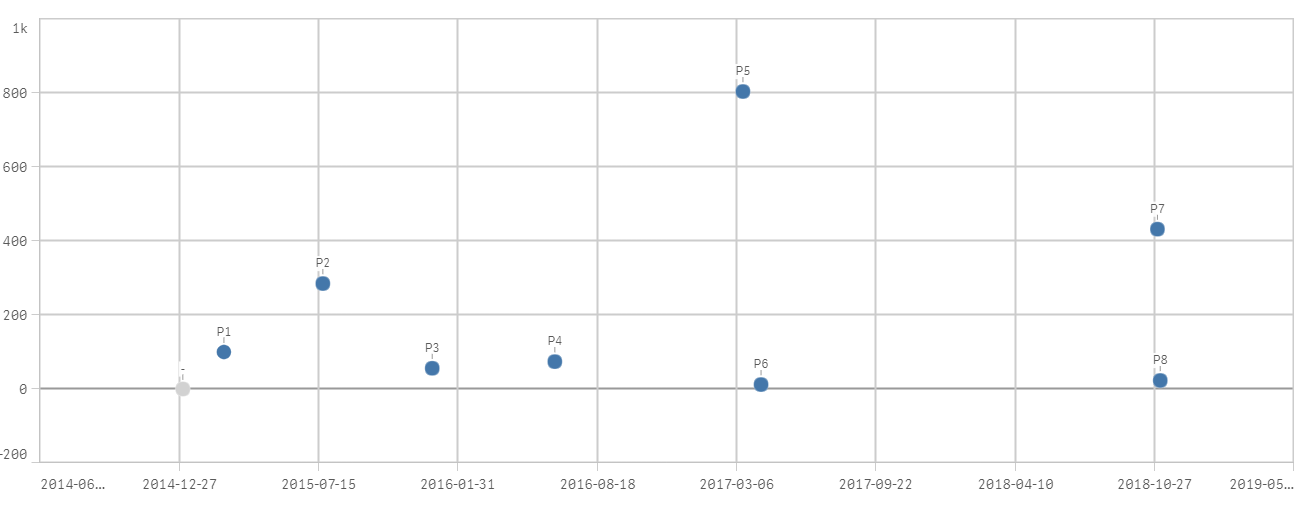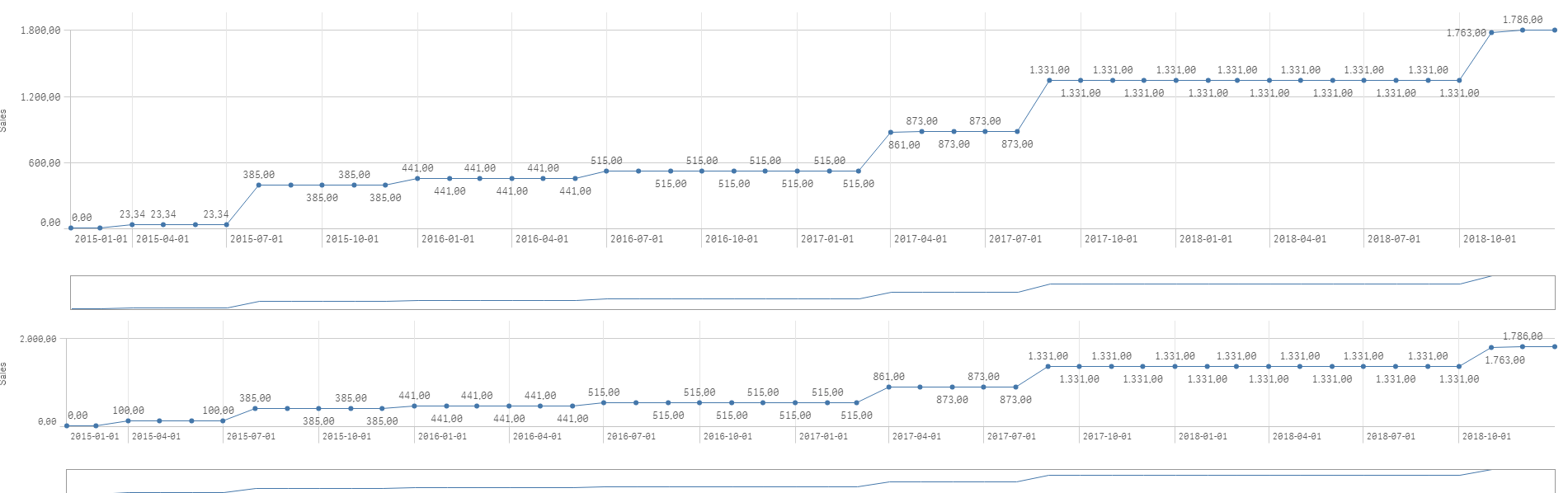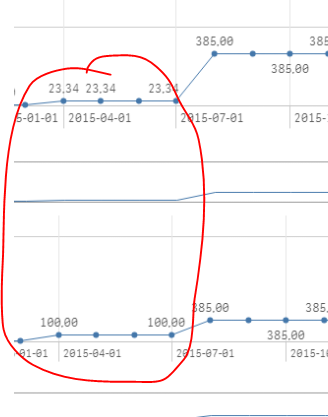# New to Qlik Sense

Discussion board where members can get started with Qlik Sense.

Highlighted
New Contributor III

## Scatter plot and Line Chart accumulated

Hi,

I need some help with two charts. On the one hand, I have a line chart that shows the accumulated amount on each end of the month date. I have created a calendar that takes me all the previous dates of each end of the month. In the graph of lines below in original currency works correctly. In the top one I added the exchange rate and I no longer get the accumulated amount.On the other hand, I have a scatter chart. The program P5 exists twice with different amounts and dates but only appears once and I would need to show two with the different amounts and dates.I attach a qvf with the 2 charts.

Thank you very much in advance.

Tags (2)
14 RepliesMVP

## Re: Scatter plot and Line Chart accumulated

May be using this

Sum(AMOUNT)*\$(vFXRates)New Contributor III

## Re: Scatter plot and Line Chart accumulated

It's not working because it dowsn't calculated well the exchange rate for all the programs. In fact the two charts ends with the same result which it's not correctMVP

## Re: Scatter plot and Line Chart accumulated

Use this as your dimension

=Dual(PROGRAM, DATE)MVP

## Re: Scatter plot and Line Chart accumulated

Some of the values are differentNew Contributor III

## Re: Scatter plot and Line Chart accumulated

only the first program calculates its exchange rate well the rest are wrong

New Contributor III

## Re: Scatter plot and Line Chart accumulated

thanks it works!MVP

## Re: Scatter plot and Line Chart accumulated

Is this not a problem in the below chart?

New Contributor III

## Re: Scatter plot and Line Chart accumulated

No, the below chart is in original currency top chart should be in euros.MVP

## Re: Scatter plot and Line Chart accumulated

What numbers do you expect to see on the top chart? We see 23.34 right now for 2015-04-01... what should it be?